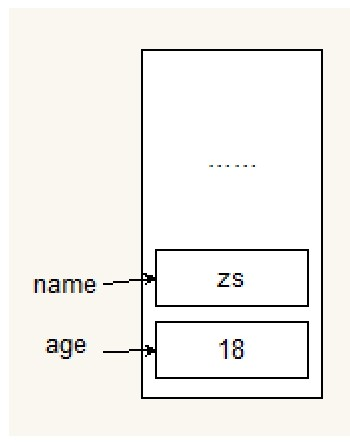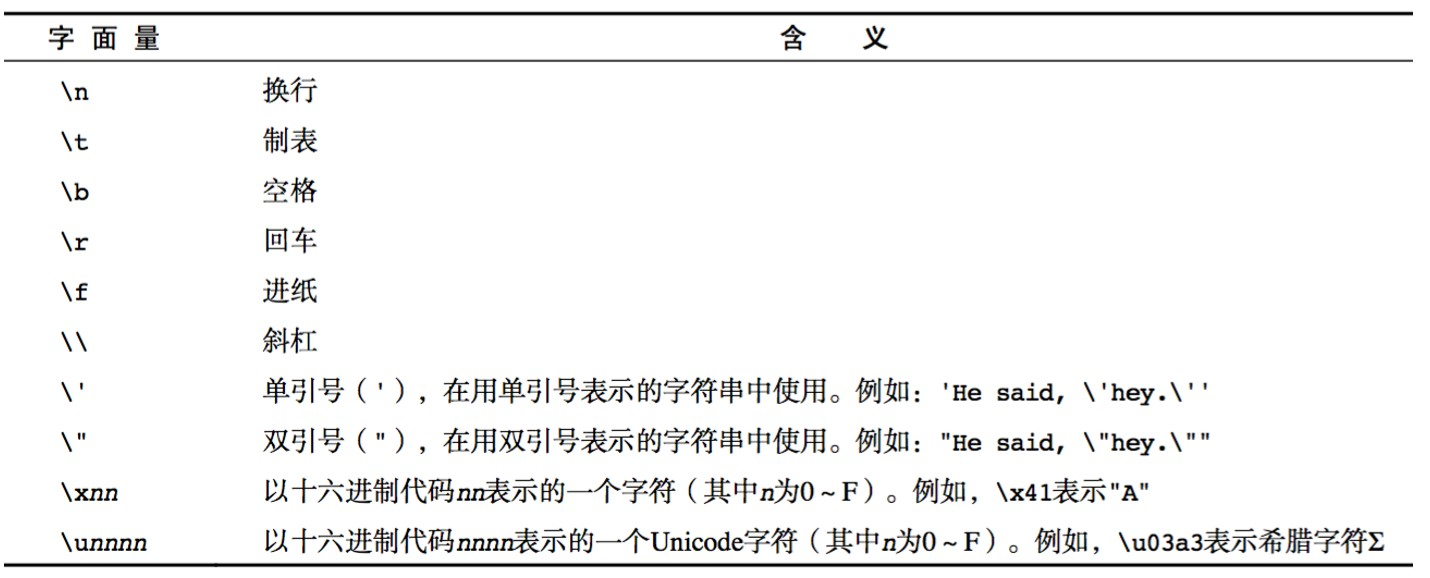## javascript基础（1）

### JavaScript介绍

JavaScript 编程语言 流程控制
Java 服务端的编程语言 JavaScript是一种运行在客户端（浏览器）的编程语言
JavaScript的解释器被称为JavaScript引擎，为浏览器的一部分，广泛用于客户端的脚本语言，最早是在HTML（标准通用标记语言下的一个应用）网页上使用，用来给HTML网页增加动态功能。

#### 最初目的

JavaScript最初目的是为了处理表单验证。

#### JavaScript现在的意义（应用场景）

1. 网页特效
2. 服务端开发（node.js）
3. 命令行工具（node.js）
4. 桌面程序（electron）
5. app（cordova）
6. 控制硬件-物联网（ruff）
7. 游戏开发（cocos2d-js）

#### JavaScript和html、css之间的区别

1. HTML：提供网页的结构，提供网页中的内容
2. Css：用来美化网页
3. JavaScript：可以用来控制网页内容，给网页增加动态效果

#### ECMAScript-JavaScript的核心

ECMA 欧洲计算机制造联合会

JavaScript的核心，描述了语言的基本语法和数据类型，ECMAScript是一套标准，定义了一种语言的标准与具体实现无关

#### DOM-文档对象类型

DOM可以把HTML看成是文档树，通过DOM提供的API可以对树上的节点进行操作

#### JavaScript的书写位置

• 写在行内
`<input type="button" value="按钮" onclick="alert('Hello World')" />`
• 写在script标签内
``````<head>
<script>
</script>
``````
• 写在外部js文件中，在页面引入
``````<script src="main.js"></script>
``````

#### 计算机组成

• 应用软件：浏览器、QQ、微信
• 系统软件：Windows、Linux、mac OSx

• 三大件：CPU、内存、硬盘—主板
• 输入设备：鼠标、键盘、手写板、摄像头
• 输出设备：显示器、打印机、投影仪

#### 变量

• 什么是变量
变量是计算机内存中存储数据的标识符，根据变量名称可以获取到内存中存储的数据
• 为什么要使用变量
使用变量可以方便的获取或者修改内存中的数据

#### 如何使用变量

• var声明变量
var age；
• 变量的赋值
var age =18；
• 同时声明多个变量
var age,name,sex;
age=10; name=‘zs’;
• 同时声明多个变量并赋值
var age = 10; name = ‘zs’;

#### 变量在内存中的存储

var age = 18；#### 命名规则和规范

• 规则-必须遵守，不遵守会报错
• 由字母、数字、下划线、\$符号组成，不能以数字开头
• 不能用关键字和保留字，例如：for,while
• 区分大小
• 规范-建议遵守的，不遵守不会报错
• 变量名必须有意义

#### 交换两个变量的值

``````<script>
// 利用临时变量来交换a,b的值
var a = 10, b = 20;
console.log('a原来是：' + a);
console.log('b原来是：' + b);
var temp = a;
a = b;
b = temp;
console.log('a现在是：' + a);
console.log('b现在是：' + b);
// 交换两个数字的值
var a = 10, b = 20;
a = a + b;   //a=30
b = a - b;   //b=10
a = a - b;   //a=20
console.log(a, b);
// 可以用^异或，因为a=1,二进制就是0001，b=2,二进制就是0010，相同为0，不同为1，所以0001和0010就是变成0011=3  a
var a = 1, b = 2;
a = a ^ b;   //a=3
console.log(a)
b = a ^ b;   //b=0001=1
console.log(b)
a = a ^ b;   //a=0010=2
console.log(a, b);
</script>
``````

#### 数据类型

##### 简单的数据类型
``````Number、String、Bollean、Undefined、Null
``````
###### Number 类型
• 数值字面量：数值的固定值的表示法 110 1024
• 进制
• 十进制 var num =9;进行算数计算时，八进制和十六进制表示的数值最终都将转换成十进制数值
• 十六进制 var num = oxA;数字序列范围：0–9以及A–F
• 八进制var num = 7;数字序列范围0–7，如果字面值中的数值超出了范围，那么前导零将忽略，后面的数值将被当做十进制解析。
• 浮点数
• 浮点数的精度问题：浮点数
var n = 5e-324; // 科学计数法 5乘以10的-324次方
浮点数值的最高精度是 17 位小数，但在进行算术计算时其精确度远远不如整数
var result = 0.1 + 0.2; // 结果不是 0.3，而是：0.30000000000000004
console.log(0.07 * 100);
不要判断两个浮点数是否相等
• 数值范围
• 最小值：Number.MIN_VALUE，这个值为： 5e-324
最大值：Number.MAX_VALUE，这个值为： 1.7976931348623157e+308
无穷大：Infinity
无穷小：-Infinity
• 数值分析
• NaN：not a number NaN 与任何值都不相等，包括他本身
• isNaN: is not a number
##### String 类型
• 字符串字面量 ‘abc’
• 转义符• 字符串长度
length属性用来获取字符串的长度
var str =‘abc’; console.log(str.length);
• 字符串的拼接
字符串的拼接用+号
console.log(‘abc’+‘dr’);
1. 两边只要有一个是字符串，那么+号就是字符串拼接功能
2. 计算机内部储存
##### undefined和Null
1. undefined表示一个声明了没有赋值的变量，变量只声明的时候默认是undefined.
2. null表示一个空，变量的值如果想为null，必须手动设置。
##### 复杂数据类型（object）
• 获取变量类型
typeof var age =18; console.log(typeof age);
在谷歌浏览器中字符串的颜色是黑色的，数值类型是蓝色的，布尔类型也是蓝色的，undefined和null是灰色的
##### 转换成字符串类型
• toString()
var num = 5; console.log(num.toString());
• String()
String存在的意义：有些值没有toString()，这个时候可以使用String()，比如：undefined和null
• 拼接字符串方式
num + “”,当+两边一个操作符是字符串类型，一个操作符是其他类型的时候，会先把其他类型转换成字符串在进行字符串拼接，返回字符串
`````` <script>
// var age = 18;
// var name = 'zs';
// var isRight = true;
// var a;
// var b = null;
// console.log(age);
// console.log(name);
// console.log(isRight);
// console.log(a);
// console.log(b);
//
// 转换成字符串类型
// 1 toString()方法
// var num = 18;
// var isRight = true;
// console.log(typeof num.toString());
// console.log(typeof isRight.toString());

// 错误的方式 null和undefined没有toString()方法
// var a = null;
// console.log(a.toString());
//
// 2 String()方法
// var num = 18;
// var isRight = true;
// console.log(String(num));
// console.log(String(isRight));

// var a = null;
// console.log(typeof String(a));
//
// 3 字符串拼接
var num = 18;
var isRight = true;
console.log(typeof(num + ''));
console.log(typeof(isRight + ''));

</script>
``````
##### 转换为数值类型
• Number()
Number()可以把任意值转换为数值，如果要转换的字符串中有一个不是数值的字符，返回NaN
• paseInt()
var num1 = parseInt(“12.3abc”); //返回12，如果第一字符是数字会解析直到遇到非数字结束
var num1 = parseInt(“abc12.3”); //返回NaN,如果第一个字符不是数字或者符号就返回NaN
• +,-0等运算
var str = ‘500’;
console.log(+str); //取正
console.log(-str); //取负
console.log(str – 0 );
``````<script>
// 1 Number()
// var str = 'abc';   //   Number(str)   -》 NaN
// var isRight = true;  //  Number(isRight) -> 1

// console.log(Number(str));
// console.log(Number(isRight));
//
// console.log(Number('123'));
// // Number() 把字符串转换成数值类型的时候，如果字符串中有一个字符不是数字，返回NaN
// console.log(Number('123abc'));
//
//
//
// 2 parseInt()  // int 整数
// var str = '123';
// var isRight = false;

// console.log(parseInt(str));
// // parseInt 无法把布尔类型转换成数值类型 返回的结果是NaN
// console.log(parseInt(isRight));

// //var s1 = 'abc';   //  parseInt(s1) -> NaN
// var s1 = '123abc';
// // parseInt 在转换字符串的时候，如果遇到数字就会转换数字，如果遇到非数字就会返回
// console.log(parseInt(s1));

// var s2 = 'abc123';  // 如果字符串中第一个字符是非数字 此时返回NaN
// console.log(parseInt(s2));

// 3 parseFloat()
// var str = '123.67';
// var isRight = true;
// console.log(parseFloat(str));
// console.log(parseInt(str));  // 返回123
// console.log(parseFloat(isRight));   // parseFloat 无法转换布尔类型  返回的是NaN

// var str = '123.12abc';
// console.log(parseFloat(str));
//
// var str = '123abc';
// console.log(parseFloat(str));
//
//
// 4 取正或者取负   + -
// var num = -5;
// console.log(num);
//
// var str = '123';
// console.log(-str);
//
// var str = '123abc';
// console.log(+str);
//
// var isOK = true;
// console.log(+isOK);

var str = '123abc';
// - 如果一边是数值类型 一边是字符串，会先把字符串转换成数值类型再进行数值的减法运算
// 如果字符串转换成数值类型失败  此时返回NaN
// console.log(str - 0);
// + 如果有一边是数值类型 一边是字符串，会先把数值类型转换成字符串 再进行字符串的拼接
console.log(str + 0);
</script>
``````
##### 转换为布尔类型
• Boolean()
0 、 ‘(空字符串)’、null、undefined、NaN 会被转换成false，其他都会换成true
`````` <script>

var str = 'abc';
var num = 123;
var a = null;
var b;  // 默认值是undefined

console.log(Boolean(str));   // true
console.log(Boolean(num));   // true
console.log(Boolean(a));     // false
console.log(Boolean(b));     // false

// 转换成false的情况：null  undefined  ''空字符串  0 NaN

console.log(Boolean(''));
console.log(Boolean(0));
console.log(Boolean(NaN));

console.log(Boolean(-1));
</script>
``````
##### 操作符

++ 自身加一
– – 自身减一

``````var num1 = 5;
++ num1;
var num2 = 6;
console.log(num1 + ++ num2);
``````

``````var num1 = 5;
num1 ++;
var num2 = 6
console.log(num1 + num2 ++);
``````

`````` <script>

// 前置++
// var num = 5;
// // ++num  表达式  先让num自身+1  然后再返回表达式的结果
// console.log(++num);

// var num1 = 7;
// // 6 + 8  = 14
// console.log(num + ++num1);
// console.log(num1);
// console.log(--num1);
//
//
// 后置++
// var num = 5;
// // num++  表达式  先返回表达式的结果num  然后再对num自身+1
// console.log(num++);
// console.log(num);

// var num1 = 7;
// // 6 + 7 =13
// //  num1: 8
// console.log(num + num1++);
// console.log(num1);
//
//
// 练习
//
// ++a   2 先执行a自身+1 ， 然后再返回表达式的结果
// ++a   3
// 5
//var a = 1; var b = ++a + ++a; console.log(b);

// 先返回表达式的结果a，再执行a自身+1
// a++    表达式 1    a = 2
// ++a    表达式 3
// 1 + 3  = 4
// var a = 1; var b = a++ + ++a; console.log(b);
//
//
//
// a++    表达式 1  a = 2
// a++    表达式 2  a = 3
// 1 + 2 = 3
// var a = 1; var b = a++ + a++; console.log(b);
//
//
// ++a   表达式 2   a = 2
// a++   表达式 2   a = 3
//   2 + 2 = 4
var a = 1;
var c = 1;
// c先运行在加1   所以b=3,a=2,c=2
// ++a 先+1再运行   a++ 先运行，再+1
var b = ++a + c++; console.log(b); console.log(a); console.log(c);
</script>
``````
##### 逻辑运算符

&& 与 两个操作数同时为true，结果为true，否则都是false
|| 或 两个操作数有一个为true，结果为true，否则为false
! 非 取反

``````<script>

// 逻辑运算符   与 &&  或 ||  非 !
// 与 --> 并且    只有两个操作数同时为true  结果才为true
// var a = false;
// var b = false;
// console.log(a && b);
//
// 或 ||   只有两个操作数同时为false  结果才为false
//
// var a = false;
// var b = false;
// console.log(a || b);
//
// !  非  取反   一元运算符
var a = false;
console.log(!a);
</script>
``````
##### 关系运算符
``````<  >  >=  <= == != === !==
==与===的区别：==只进行值得比较，===类型和值同时相等，则相等

var result = '55' == 55;  	// true
var result = '55' === 55; 	// false 值相等，类型不相等
var result = 55 === 55; 	// true
``````
`````` <script>

// 关系运算符
// <  >  >=  <=
//
// var a = 10;
// var b = 5;
// console.log(a > b);
// console.log(a < b);

// == !=
// console.log(a == b);
// console.log(a != b);
// === 相等判断 !==  不等
var a = 10;
var b = 10;
// == 判断的是变量的值是否相等
// console.log(a == b);
// === 判断值和类型都一致才返回true
console.log(a === b);

</script>
``````
##### 赋值运算符
``````=   +=   -=   *=   /=   %=

var num = 0;
num += 5;	//相当于  num = num + 5;
``````
##### 运算符的优先级

1. （）优先级最高
2. 一元运算符 – 、++
3. 关系运算符 先乘除除余后加减
4. 关系运算符 > 、>=、<、<=
5. 相等运算符 `== 、！==、===、！==`
6. 逻辑运算符 先&&后||
7. 赋值运算符